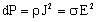# Energies and powers (magnetic system)

## Introduction

This section discusses the stored energy and the power dissipated in a magnetic system.

## Electro-magnetic energy: definition (IEC)

The electromagnetic energy is the energy associated with the presence of an electromagnetic field.

Note: In a linear medium the electromagnetic energy is given by the volume integral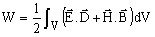where; E, D, H and B are the four vector quantities determining the electromagnetic field.

## Starting from the Poynting vector …

It is convenient to define the electromagnetic energy by means of the Poynting vector: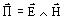(according to …)

The vector analysis associated with the Maxwell equations then gives: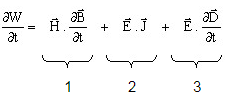1. The first term describes the energy stored as magnetic energy
2. The second term describes the energy dissipated by the Joule effect
3. The third term describes the energy stored as electric energy

## Stored magnetic energy

To create a magnetic field in a region we must supply some energy, which will be stored as magnetic energy.

The volume density of the stored magnetic energy can be expressed by means of the vector quantities B and H in the relationship: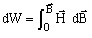which in a linear homogeneous isotropic region can be equally written as: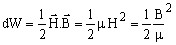## Dissipated power / Power losses by Joule effect

The volume density of the dissipated power (or the volume density of power losses by Joule effect) is expressed by means of E and J in the relationship: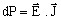In a linear homogeneous isotropic region the corresponding equality is: